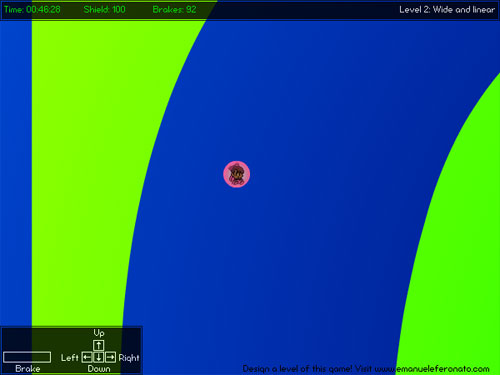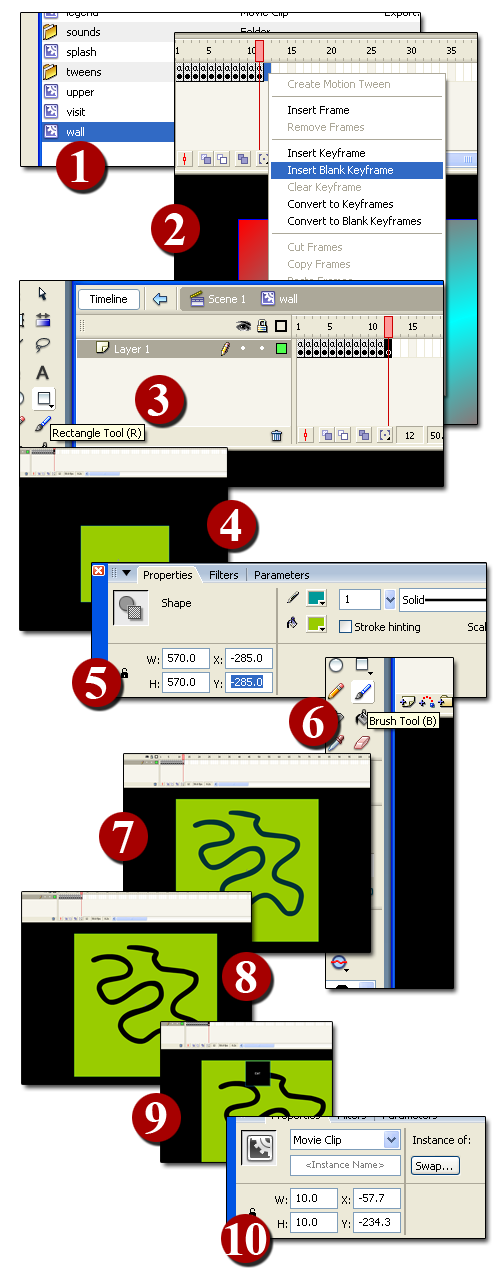# Tunnelball: design a level for this flash game

I want you all to play TUNNELBALL, a complete flash game I developed using most of the topics covered in tutorials 1 to 5.3Moreover, I am releasing the full source code and I invite you all to contribute to the sequel of this game by designing one or more leves for the sequel I am planning. I will teach you how to do it in this tutorial.

But first of all play the game.

The source code is commented at its inside and there isn’t anything you haven’t already seen.

Let’s examine it:

All the actionscript is located in the 1st frame

```// TUNNELBALL V0.1 BETA
// By Emanuele Feronato
// www.emanueleferonato.com
// visit my blog and find my tuotorials
// attaching movies
attachMovie("bg", "bg", 1, {_width:5700, _height:5700});
attachMovie("explosion", "explosion", 2, {_x:284, _y:209, _visible:false});
attachMovie("kira", "kira", 3, {_x:284, _y:209});
attachMovie("wall", "wall", 4, {_width:5700, _height:5700});
attachMovie("upper", "upper", 5, {_x:300, _alpha:80});
attachMovie("count_down", "count_down", 6, {_x:0, _y:0, _alpha:80});
attachMovie("legend", "legend", 7, {_x:0, _y:391, _alpha:80});
attachMovie("game_over_button", "game_over_button", 8);
attachMovie("splash", "splash", 9, {_x:440, _y:113, _alpha:80});
attachMovie("visit", "visit", 10, {_x:250, _y:433});
// the game_over_button properties aren't set in the attachmovie declaration as I did
// with the others movieclips because it didn't work. Seems that button's properties
// cannot be declared "on the fly".
game_over_button._visible = false;
game_over_button._x = 284;
game_over_button._y = 209;
game_over_button.onRelease = function() {
getURL('http://www.emanueleferonato.com');
};
// attaching sounds
hit_sound = new Sound();
hit_sound.attachSound("sound_hit");
gameover_sound = new Sound();
gameover_sound.attachSound("gameover");
// player's starting positions
start_x = new Array(240, 240, 240, -2100, 2690, -1800, 1400, 2700, 200, 2700, 250);
start_y = new Array(0, 350, 0, 2500, 2800, 2500, 2000, 2600, 600, 2750, 190);
// level names
level_names = new Array("Intro", "Wide and linear", "Not so wide...", "Long but straight", "Crazy serpentine", "Use your brakes", "Intestine", "The bunker", "Back and forth", "Impossible: give up", "Congratz!!");
init_game();
// main function
kira.onEnterFrame = function() {
if (kira_playing) {
// timer
if (level>1) {
elapsed_time = getTimer()-start_time;
}
time_left = countdown-elapsed_time;
if (time_left<45000) {
count_down.time_left.textColor = 0xffff00;
}
if (time_left<30000) {
count_down.time_left.textColor = 0xff8000;
}
if (time_left<15000) {
count_down.time_left.textColor = 0xff0000;
}
count_down.time_left.text = time_to_string(time_left);
// detecting keys pressed
if (Key.isDown(Key.LEFT)) {
xspeed = xspeed-power;
}
if (Key.isDown(Key.RIGHT)) {
xspeed = xspeed+power;
}
if (Key.isDown(Key.UP)) {
yspeed = yspeed-power*upconstant;
}
if (Key.isDown(Key.DOWN)) {
yspeed = yspeed+power*upconstant;
}
if ((Key.isDown(Key.SPACE)) and (brakes>0)) {
yspeed = yspeed/2;
xspeed = xspeed/2;
// I don't want the player to lose brakes in the 1st level
if (level>1) {
brakes--;
count_down.brake_left.textColor = 0x00ff00;
if (brakes<75) {
count_down.brake_left.textColor = 0xffff00;
}
if (brakes<50) {
count_down.brake_left.textColor = 0xff8000;
}
if (brakes<25) {
count_down.brake_left.textColor = 0xff0000;
}
count_down.brake_left.text = "Brakes: "+brakes;
}
}
// speed calculation
xspeed = (xspeed+wind)*friction;
yspeed = yspeed+gravity;
if (xspeed>0) {
this.kira.gotoAndStop(1);
} else {
this.kira.gotoAndStop(2);
}
// oh no!!! kira dies!!!
if ((energy<1) or (countdown-elapsed_time<1)) {
kira_die();
}
// screen update
wall._y -= yspeed;
wall._x -= xspeed;
bg._y -= yspeed;
bg._x -= xspeed;
// collision
if (wall.hitTest(this._x, this._y, true)) {
if (!wall.end.hitTest(this._x, this._y, true)) {
// if the level is not the 1st, the player lose energy
if (level>1) {
energy -= Math.round(Math.sqrt(xspeed*xspeed+yspeed*yspeed));
count_down.energy_left.textColor = 0x00ff00;
if (energy<75) {
count_down.energy_left.textColor = 0xffff00;
}
if (energy<50) {
count_down.energy_left.textColor = 0xff8000;
}
if (energy<25) {
count_down.energy_left.textColor = 0xff0000;
}
count_down.energy_left.text = "Shield: "+energy;
}
xspeed = 0;
yspeed = 0;
hit_sound.start();
wall._y = old_y;
wall._x = old_x;
bg._y = old_y;
bg._x = old_x;
} else {
xspeed = 0;
yspeed = 0;
splash._visible = false;
if (level>1) {
energy += 100;
brakes += 100;
start_time += 60000;
} else {
start_time = getTimer();
}
level++;
count_down.energy_left.text = "Shield: "+energy;
count_down.brake_left.text = "Brakes: "+brakes;
count_down.energy_left.textColor = 0x00ff00;
count_down.time_left.textColor = 0x00ff00;
count_down.brake_left.textColor = 0x00ff00;
position_bg(level);
}
} else {
old_x = wall._x+2*xspeed;
old_y = wall._y+2*yspeed;
}
} else {
// once the game is over
if (Key.isDown(Key.SPACE)) {
init_game();
}
}
};
function time_to_string(time_to_convert) {
elapsed_hours = Math.floor(time_to_convert/3600000);
remaining = time_to_convert-(elapsed_hours*3600000);
elapsed_minutes = Math.floor(remaining/60000);
remaining = remaining-(elapsed_minutes*60000);
elapsed_seconds = Math.floor(remaining/1000);
remaining = remaining-(elapsed_seconds*1000);
elapsed_fs = Math.floor(remaining/10);
if (elapsed_hours<10) {
hours = "0"+elapsed_hours.toString();
} else {
hours = elapsed_hours.toString();
}
if (elapsed_minutes<10) {
minutes = "0"+elapsed_minutes.toString();
} else {
minutes = elapsed_minutes.toString();
}
if (elapsed_seconds<10) {
seconds = "0"+elapsed_seconds.toString();
} else {
seconds = elapsed_seconds.toString();
}
if (elapsed_fs<10) {
hundredths = "0"+elapsed_fs.toString();
} else {
hundredths = elapsed_fs.toString();
}
return "Time: "+minutes+":"+seconds+":"+hundredths;
}
function position_bg(level) {
wall.gotoAndStop(level);
count_down.level_name.text = "Level "+level+": "+level_names[level-1];
wall._x = start_x[level-1];
wall._y = start_y[level-1];
bg._x = start_x[level-1];
bg._y = start_y[level-1];
}
function kira_die() {
gameover_sound.start();
kira._visible = false;
explosion._visible = true;
explosion.gotoAndPlay(1);
game_over_button._visible = true;
xspeed = 0;
yspeed = 0;
kira_playing = 0;
}
function init_game() {
level = 1;
elapsed_time = 0;
position_bg(level);
yspeed = 0;
xspeed = 0;
wind = 0.00;
power = 0.65;
gravity = 0.1;
upconstant = 0.75;
friction = 0.99;
energy = 100;
brakes = 100;
kira_playing = 1;
start_time = getTimer();
countdown = 60000;
kira._visible = true;
explosion._visible = false;
game_over_button._visible = false;
splash._visible = true;
with (count_down.brake_left) {
text = "Brakes: "+brakes;
textColor = 0x00ff00;
}
with (count_down.energy_left) {
text = "Shield: "+energy;
textColor = 0x00ff00;
}
with (count_down.time_left) {
textColor = 0x00ff00;
}
}```

There is nothing more to comment in my opinion, but if someone will ask for some explaination, I will be happy to spend some more words on it.

The topic I want to talk about now, is:

How did I design a level?

Levels are squares 570 pixels wide and 570 pixels tall, that are enlarged by 10 when in game.

In these squares, in the same level, I started drawing the "track" with the brush tool, and then I removed the drawing.

Let's see how: let's suppose we want to design the 11th level of the game: it's easy to follow these 10 steps:1: Select the wall movieclip by double clicking on it in the library window

2: Create a new empty keyframe in the 11th frame

3: Select the rectange tool

4: Draw a rectangle

5: Define its width and height as 570 pixels and position it at (-285,-285)

6: Select the brush tool

7: Draw the "track" over the rectangle

8: Delete the brush shape: you will delete a portion of the rectangle too!

9: Place the end movieclip on the same level

10: Since the movieclip will be resized by 10x, scale down the end movieclip at x=10, y=10

Congratulations!!

You have just created a track!

To play it, just add a value to the arrays at lines 31, 32 and 34 and you are ready to go!

I am planning a sequel of Tunnelball (of course we will make it together as I will describe any new feature with a tutorial), so I need some tracks you will design!! Unleash your fantasy, design some nice tracks, save the .fla and send it to info@emanueleferonato.com.

Your name, along with your personal webpage, will be listed in the credit section of the game! And in this site too!!

214 GAME PROTOTYPES EXPLAINED WITH SOURCE CODE
// 1+2=3
// 10000000
// 2 Cars
// 2048
// Avoider
// Ballz
// Block it
// Blockage
// Bloons
// Boids
// Bombuzal
// Breakout
// Bricks
// Columns
// CubesOut
// Dots
// DROP'd
// Dudeski
// Eskiv
// Filler
// Fling
// Globe
// HookPod
// Hundreds
// InkTd
// Iromeku
// Lumines
// Magick
// MagOrMin
// Maze
// Memdot
// Nano War
// Nodes
// o:anquan
// Ononmin
// Pacco
// Phyballs
// Platform
// Poker
// Pool
// Poux
// Pudi
// qomp
// Racing
// Renju
// SameGame
// Security
// Sling
// Slingy
// Sokoban
// Splitter
// Sproing
// Stack
// Stringy
// Sudoku
// Tetris
// Threes
// Toony
// Turn
// TwinSpin
// vvvvvv
// Wordle
// Worms
// Yanga
// Zhed
// zNumbers